Next: Sidebands Up: Experimental Approach and Equipment Previous: Finding the Depolarizing Resonance   Contents

## Resonance Mirror

In the last chapter we mentioned the fact that only the fractional part of the spin tune is measurable. Similar to the fact that a traveler going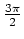around a circle ends at the same spot his fellow does when going around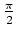in the other direction, we can't be a priori sure when observing a resonant'' frequency that this isn't just the mirror frequency of the resonance. According to the Nyquist Theorem we can only measure how much below or above we are from the half-integer, but not on which side or in mathematical terms, does the measured frequency correspond to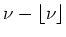or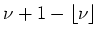. For example the frequency 500 kHz corresponds to a beam energy of 2.372 GeV but also to the mirror energy at 2.475 GeV; this ambiguity is resolved in the following way:

The energy of the beam can be slightly varied using a different RF main frequency. At SLS the RF main frequency is 500 MHz. Beam energy and RF main frequency are related by the momentum compaction factor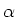: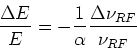(52)

Therefore a change in the RF main frequency will lead to a change in beam energy. If the RF main frequency is changed to a lower value, the beam energy will rise. If the resonant frequency increases as well it is the real'' resonance; if however the resonant frequency decreases, it is the mirror resonance and vice-versa. Thus this procedure always requires a second measurement of the resonant frequency.Next: Sidebands Up: Experimental Approach and Equipment Previous: Finding the Depolarizing Resonance   Contents
Simon Leemann
2002-03-15